## Write a repeating decimal that is between 9/7 and 10/7. Justify your answer.

Question

Write a repeating decimal that is between 9/7
and 10/7.

in progress 0
5 months 2021-09-05T07:59:34+00:00 1 Answers 0 views 0

## Answers ( )

1. Given:

Two numbers are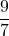and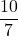.

To find:

A repeating decimal that is betweenand.

Solution:

Using calculator, we get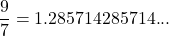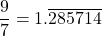and,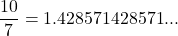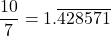Now, the repeating decimal that is betweenandbe x. So,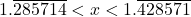On analyzing the numbers to hundredth places, we get 1.28 < 1.33 < 1.42, therefore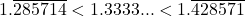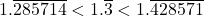And we know that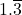is the decimal form of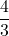.

Therefore, the required number is.